# 基于智能压实技术对三种土路基的压实度试验研究Experimental Study on Compaction Degree of Three Kinds of Soil Subgrade Based on Intelligent Compaction Technology

DOI: 10.12677/HJCE.2018.73049, PDF, HTML, XML, 下载: 809  浏览: 2,315  科研立项经费支持

Abstract: A model with a filling body as a dispersion and the response signal processing model of vibratory roller are established, which make a research analysis for three kinds of soil subgrade materials, by using MGYS-III. Correlation analysis and regression analysis were carried out on the compactness values obtained through CMV and perfusion method.

1. 前言

2. 压路机在碾压过程中对填筑体建模求解和分析

2.1. 填筑体为离散体——“质量块–弹簧–阻尼”模型

2.1.1. “质量块–弹簧–阻尼”模型建立与解析

2.2. 振动压路机响应信号处理模拟——谐波比模型

2.2.1. 振动力学模型系统分析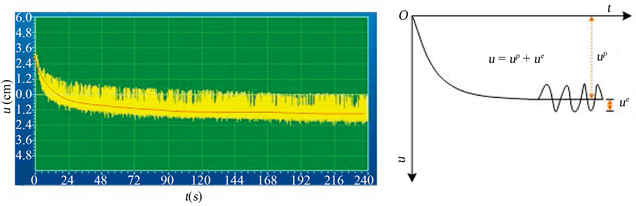Figure 1. Deformation process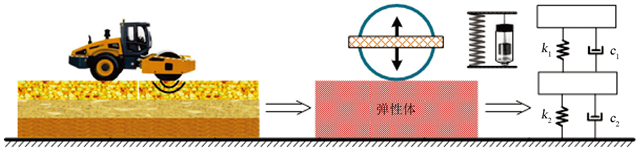Figure 2. Discrete body of filling body—“mass block-spring-damping” modelFigure 3. Two-degree-of-freedom system and single-degree-of-freedom system model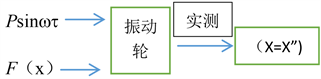Figure 4. Oscillation dynamics model system diagram

2.2.2. 建立振动响应和抗力的相关关系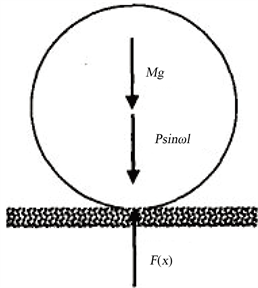Figure 5. Force diagram of excitation system

3. MGYS-Ⅲ智能压实控制系统实验方法

3.1. 试验工况

3.2. MGYS-III系统反映路基的填料和压实状况的分析

3.2.1. 对填料的含水量及压实度的分析

3.2.2. 对填料的均匀度的分析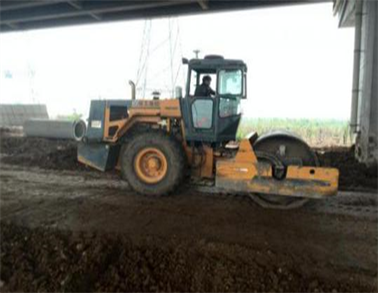Figure 6. High water content in this section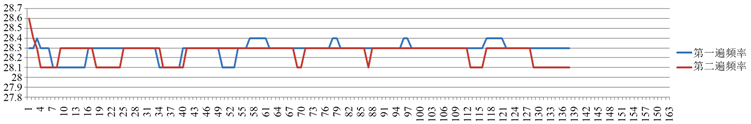(a)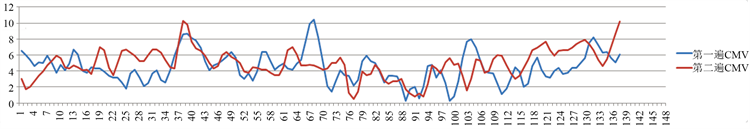(b)

Figure 7. (a) MGYS-III feedback vibration frequency; (b) CMV sequence diagram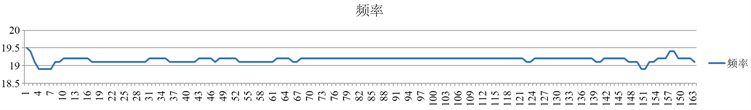Figure 8. Vibration frequency obtained by feedback of MGYS-III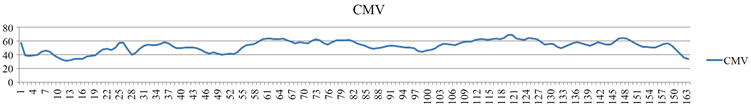Figure 9. CMV time seriesTable 1. Vibration parameters of roller at this stageTable 2. Vibration parameters of roller at this stage

3.2.3. 对压实的均匀性的分析

4. 智能压实控制指标CMV与压实度相关校验

4.1. 连续压实检测结果选取

4.1.1. 现场取点数据整理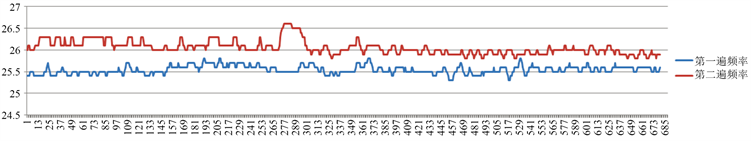Figure 10. Vibration frequency obtained by feedback of MGYS-III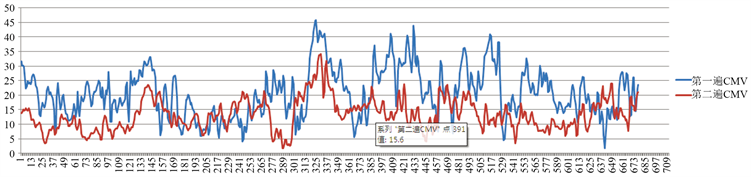Figure 11. CMV sequence diagram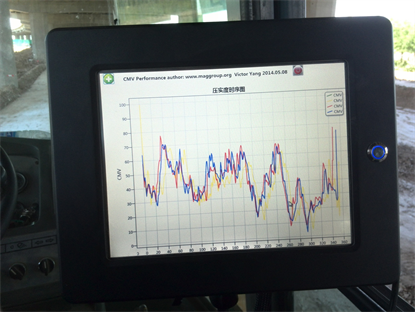Figure 12. Control terminal displays CMV curve

4.1.2. 曲线拟合的选择

$Nn\left(x\right)={a}_{0}+{a}_{1}\left(x-{x}_{0}\right)+{a}_{2}\left(x-{x}_{0}\right)\left(x-{x}_{1}\right)+\cdots +{a}_{n}\left(x-{x}_{0}\right)\cdots \left(x-{x}_{n}-1\right)$

4.2. 智能压实压实度与CMV值得线性计算

${\gamma }_{12}=\frac{\underset{k=1}{\overset{n}{\sum }}\left({\chi }_{1k}-{\stackrel{¯}{\chi }}_{1}\right)\left({\chi }_{2k}-{\stackrel{¯}{\chi }}_{2}\right)}{\sqrt{\underset{k=1}{\overset{n}{\sum }}{\left({\chi }_{1k}-{\stackrel{¯}{\chi }}_{1}\right)}^{2}\underset{k=1}{\overset{n}{\sum }}{\left({\chi }_{2k}-{\stackrel{¯}{\chi }}_{2}\right)}^{2}}}$

4.3. 智能压实压实度与CMV值的回归模型计算以及校验预测4.3.1回归模型计算

$\begin{array}{l}y=a+bx\\ a=\stackrel{¯}{y}-b\stackrel{¯}{x};\text{\hspace{0.17em}}\text{\hspace{0.17em}}b=\frac{\underset{i=1}{\overset{n}{\sum }}\left({x}_{i}-\stackrel{¯}{x}\right)\left({y}_{i}-\stackrel{¯}{y}\right)}{\underset{i=1}{\overset{n}{\sum }}{\left({x}_{i}-\stackrel{¯}{x}\right)}^{2}}\end{array}$

x——灌砂法测得的结果，普通填料为地基系数，化学改良土为压实系数；

y——CMV；

${x}_{i}$${y}_{i}$ ——x和y分别代表智能压实压实度和CMV值，其中 $i=1,2,3,4,\cdots ,n$ ，代表数据中的数量；

a，b——回归系数。x——灌砂法测得的结果，普通填料为地基系数，化学改良土为压实系数；

y——CMV；

${x}_{i}$${y}_{i}$ ——x和y分别代表智能压实压实度和CMV值，其中 $i=1,2,3,4,\cdots ,n$ ，代表数据中的数量；

c，d——回归系数。

4.3.1. 线性回归模型校验与预测Table 3. Linear regression model check and prediction table

5. 结论

  Q/CR9210-2015. 铁路路基填筑工程连续压实控制技术规程[S].  徐光辉. 中国交通领域连续压实控制发展概述[J]. 建设机械技术与管理, 2014(8): 43-45.  董勤建, 张永进, 管照龙. 灌水法检测煤矸石压实度的影响因素[J]. 甘肃科技, 2011(16).  王奎. 压实度检测与级配碎石压实质量控制[D]. 西安: 长安大学, 2010.  崔树华, 汪学斌, 周峰. 压实度实时检测及智能压实技术的发展现状. 筑路机械与施工机械化, 2013, 30(3).  林冬. 振动波衰减与压实度关系的试验探索[D]. 西安: 长安大学, 2011.  Intelligent Compaction Technology for HMA Applications. Generic-IC Specification for HMA. June 2011.  Adam, D. and Kopf, E. (2000) Theoretical Analysis of Dynamically Loaded Soils. European Workshop Compaction of Soils and Granular Materials, 3-16.  US Department of Transportation FHWA. Tech Brief-Intelligent Compaction Executive Summary. Summer 2013.  杜文雅. 智能压实中的GPS测距技术研究[D]: [硕士学位论文]. 太原: 太原科技大学, 2014.